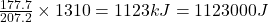## How many joules of energy are required to vaporize 13.1 kg of lead at its normal boiling point?

Question

How many joules of energy are required to vaporize 13.1 kg of lead at its normal boiling point?

in progress 0
5 months 2021-09-05T10:12:23+00:00 1 Answers 3 views 0

1. Answer: 1123000 Joules of energy are required to vaporize 13.1 kg of lead at its normal boiling point

Explanation:

Latent heat of vaporization is the amount of heat required to convert 1 mole of liquid to gas at atmospheric pressure.

Amount of heat required to vaporize 1 mole of lead =  177.7 kJ

Molar mass of lead = 207.2 g

Mass of lead given = 1.31 kg = 1310 g       (1kg=1000g)

Heat required to vaporize 207.2 of lead = 177.7 kJ

Thus Heat required to vaporize 1310 g of lead =Thus 1123000 Joules of energy are required to vaporize 13.1 kg of lead at its normal boiling point